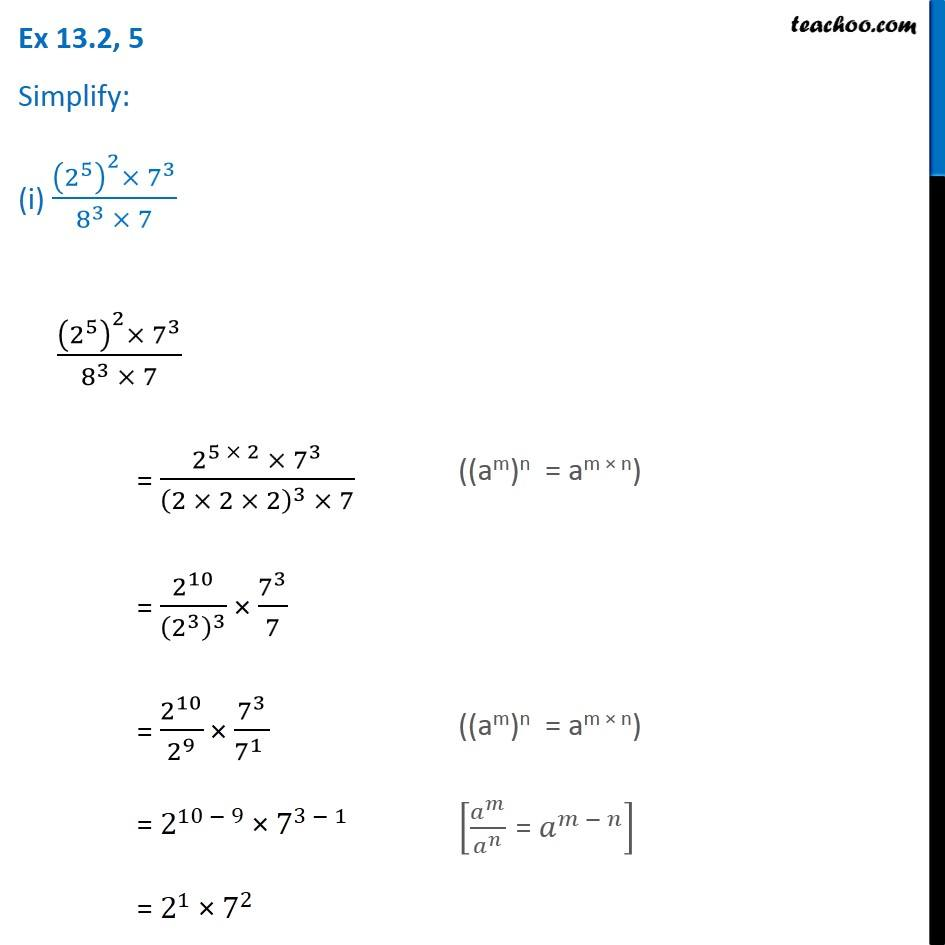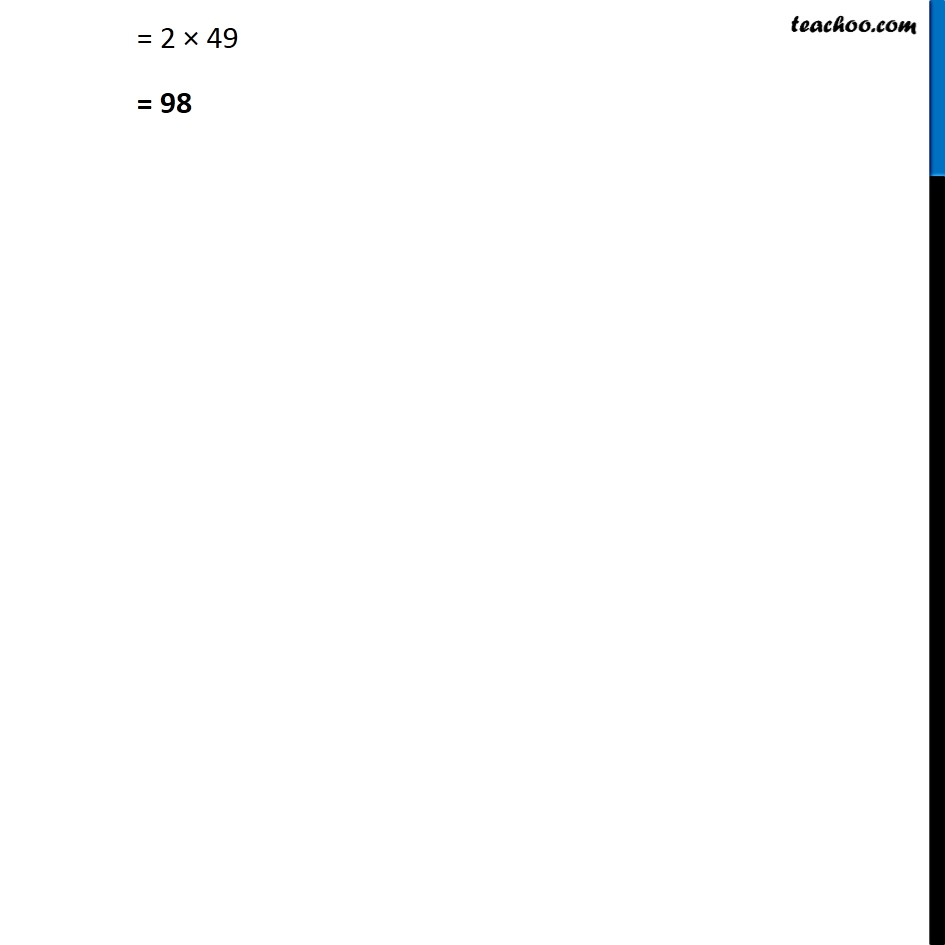1. Chapter 13 Class 7 Exponents and Powers
2. Concept wise
3. Law of exponents

Transcript

Ex 13.2, 5 Simplify: (i) ((2^5 )^2×〖 7〗^3)/(8^3 × 7) ((am)n = am × n) = 2^10/(2^3 )^3 × 7^3/7 ((am)n = am × n) = 2^(10 − 9) × 7^(3 − 1) [𝑎^𝑚/𝑎^𝑛 " = " 𝑎^(𝑚 − 𝑛) ] = 2^1 × 7^2 = 2 × 49 = 98